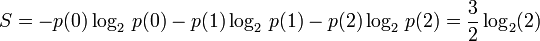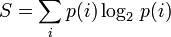Numb3rs
Season 5
Episode 11: The Arrow of Time

In this episode, the notion of time is repeatedly referenced.  While time is an extremely intuitive notion to we humans, we have a hard time explaining what it is.  To paraphrase Stephen Hawking, why do we remember the past but not the future?  Why do we perceive time as moving only from past to future?

Stephen Hawking posited the existence of three notions (arrows) of time:
1. Psychological time.  This corresponds to the human perception of time.  Things we remember are in the past.  We know we have moved forward in time if we can remember more things "now" than we did in a "previous" moment.  We seem to be capable of remembering whether we knew something "in the past."  We seem to be able to predict, with some degree of accuracy, events which happen in the future, yet we also lack complete knowledge of the future.
2. Entropic time.  This notion of time corresponds to the perceived law of nature that the universe seems to prefer moving from a state of order toward a state of disorder.  We will define exactly what entropy is later, but the basic idea is that while we commonly see a falling coffee cup shatter into pieces when it hits the floor, we never witness pieces of a broken glass lying on the floor reform into a coffee cup.
3. Cosmological time.  We won't discuss this framework of time very much, but this arrow moves forward when the universe is an inflationary state and backward when the universe is in a deflationary state.

What is entropy?

We will define entropy through a simplified example.  Suppose 2 people watch an extremely average film.  They are asked to rate the film as good or bad by giving a thumbs up or a thumbs down.  The film is so average that both people have a hard time deciding, and, ultimately, they have a 50% chance of deciding they like or dislike the film.  We define the state of our group to be the number of thumbs up.  Thus there are 3 states (remember that we could have zero thumbs up).  We will denote with p(i) the probability of having exactly i thumbs up.  p(0)=1/4, p(1)=2/4, and p(2)=1/4.  We define the entropy S as follows:If there were three people, one would simply continue the sum to include -p(3)ln p(3), and so on.  In general, the entropy can defined this way for any probability: one simply takes the sum of -p(x)ln p(x) over all x for which the probability is defined (of course one needs to neglect terms for which p(0)=0).

What does entropy mean?

As we stated above, entropy can be defined for any probability p.  Specifically,Entropy is an important quantity in what is called information theory.  Suppose that, during a war, it becomes important to transmit information using a code so that enemies cannot read it.  One of the simplest ways to do this is to assign to every letter a sequence of 0s and 1s in such a way that there is no possibility of confusion (i.e. there is a unique way to decode every message).  One way to do this is:

a - 1
b - 01
c - 001
d - 0001
e - 00001
f - 000001
g - 0000001
h - 00000001
i - 000000001
j - 0000000001
k - 00000000001
l - 000000000001
m - 0000000000001
n - 00000000000001
o - 000000000000001
p - 0000000000000001
q - 00000000000000001
r - 000000000000000001
s - 0000000000000000001
t - 00000000000000000001
u - 000000000000000000001
v - 0000000000000000000001
w - 00000000000000000000001
x - 000000000000000000000001
y - 0000000000000000000000001
z - 00000000000000000000000001

For example, the name Shannon would be:

0000000000000000001000000011000000000000010000000000000100000000000000100000000000001

There cannot be any confusion as to how this code would be interpreted: the only thing we need to check is the number of zeros between the ones.  However, this is an extremely inefficient way to encode a message.  For example, the letter s is much more frequent in the English language than j.  So we should probably assign the letter s a shorter code so that our messages are shorter.  Even if we assigned the shortest code to the most frequently occurring letters in English, this code is still probably not a very inefficient method for coding our messages.  Can you come up with a better one?

This begs the question: what is the best we can do?  This isn't very well-posed question.  Suppose we know the probability that each letter of the alphabet appears in the English language.  We assign to each letter a sequence of 0s and 1s of length l(letter) in such a way that we can decode the message.  What is the average length of the 26 codes produced (27 if we include a character for spaces)?  More specifically, what is the smallest expected code length possible?  The expected code length is produced by computing the length of the code for a letter multiplied by the probability of that letter appearing in English and then adding all these quantities together.  Expected length is sometimes also referred to as the weighted average of the lengths.

If that problem does not give you pause, suppose that instead of the alphabet we take the collection of English letters along with all pairs of English letters (or all pairs and triples of letters, for example).  Using the information collected by the Google Books project, we compute the frequency of each one and two letter combination that appears in the collected works of all English authors.  What is the smallest possible expected length of such a code?

There is a famous result in mathematics that the smallest expected code length lies between S and S+1.  So if we think of a probability as representing the likelihood of a particular letter being chosen, the entropy represents the difficulty of efficiently encoding that information into binary (0s and 1s).

It is easy to show using calculus (specifically, the method of Lagrange multipliers) to show that for n letters, the probability distribution which has greatest entropy is the uniform distribution (meaning that the probability of any letter is 1/n).  This makes intuitive sense since if one is given a particular code, the only real leeway one gets is if some letters, like the letter z in English, can be assigned longer codes so that more common letters, like e, can be assigned shorter codes.  If every letter is equally likely, this trick won't work.

How does this relate to real life?

Well, first, one needs to relate entropy to physics.  The typical example in thermodynamics is a box of gas.  The box has a barrier which splits the box into two halves, and the entirety of the gas lies on one side of the box.  The barrier is then removed.  We label each atom of gas with a 0 if it lies in the left side of the box and a 1 if it lies in the right side.  We define the state of the gas at any particular moment as the sequence of 0s and 1s generated by the labels of all the atoms.  We then ask what the probability of the gas lying in any particular state is.  We then plug this probability into the definition of entropy.

Typically, the number of molecules in a particular quantity of gas is measured in units called moles which consists of a number of particles on the order of 10^23, so it is not feasible to actually write this probability down.  Unphased by this, physicists still asked how the entropy should change over time.  The result, known as the second law of thermodynamics, states that the entropy of a closed system can only increase.  A closed system is one that cannot thermodynamically interact with anything but itself (specifically, matter and energy cannot flow out of such a system).  In fact, physicists went further.  They argued that the entropy should tend to its maximum.  This means that the probability of the system lying in any particular state tends to be uniform (i.e. all states are equally likely) as time goes on.

In terms of our example, this means that, over time, the sequences of zeros and ones will all be eventually be equally likely.  But this means, with extremely high probability, that gas will not lie on one side of the box: for one mole of gas, the probability of the sequence having only ones or zeros is on the order of 2^(-10^23) which any calculator you ask will tell you is exactly zero.  One can compute the probability of some number of atoms lying more on the left versus the right, and for small numbers the probability is high.  But the probability of any significantly higher (i.e. relatively easily detectable) proportion of the gas lying on one side versus the other is basically zero.  This is what we expect: when the gas is released, the molecules tend to fill up the space so that neither side of the box will have more molecules than the other.  Of course, it is possible force the gas into a particular state.  For example, one can chill water vapor into water and then the water will move to the bottom of the box.  However, in order to do this one must expend energy (through a refrigerator, for example).  In doing so, one increases the temperature of the air surrounding the refrigerator.  One can show that this, in turn, increases the entropy of the surrounding air in such a way that the entropy of the fridge-air combined system actually increases.

How does this relate to time?

Hawking argued that the first two notions of time are the same.  Our brains (at the very least computers) are made up of pairs of neurons.  Memories are formed by these pairs of neurons orienting themselves in a particular way.  In order to create a memory, i.e. to cause our neurons to orient in a particular fashion, requires energy which results in our body heating up a little bit.  And so the total entropy increases.  Thus our memory increases only when entropy increases, and so the two arrows must point in the same direction.